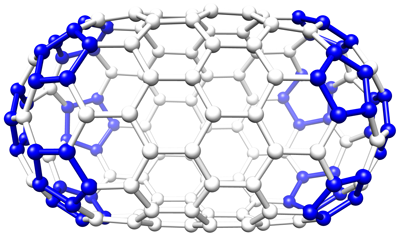## Polyhedra

A polyhedral model is composed of components (usually called vertices) and links (also called edges), but also has polygons formed by rings of links, and usually has specific rules for vertex or polygon valency. The following list defines specific types of polyhedra and polyhedral concepts:

• A polyhedron typically has a fixed vertex valency, or a fixed polygon order.
• The dual of a polyhedron switches the locations of vertices with polygons, such that a polyhedron with fixed valency becomes a polygon with fixed polygon order.
• A deltagraph is a polyhedron with triangular polygons.
• A fullerene is a polyhedron with trivalent vertices, 12 pentagons and a variable number of hexagons.
• A polygon is considered closed when it either has the same valency for all vertices, or the same order for all polygons.

The easiest way to visualize polyhedra is using UCSF Chimera and converting the model to the Chimera marker model format (cmm):

The options for specifying the component and link radii may be necessary to produce reasonable display sizes for these elements.

## Generating polyhedra

Bsoft offers various ways to generate polyhedra (e.g., Heymann et al., 2008).

### Cylindrical algorithm

A cylindrical polygon can be a tube that is open at the ends, or closed with a specific cap type. The pentagonal type adds a semi-icosahedral cap to each end, while the hexagonal type adds a six-fold cap at each end. First, a deltagraph is generated as a cylinder with flat ends:

It is then converted to its dual to give a fullerene:

bpoly -verb 1 -dual -out loz_dual.cmm loz.cmm

This is still distorted and has to be regularized:

bpoly -verb 1 -reg 10000 -linklen 1 -Klink 0.1 -Kpolyangle 0.01 -Kpolyplane 0.0001 -out loz_reg.cmm loz_dual.cmm

The pentagons can then be highlighted by color:

bmodcol -verb 1 -polygons 5 -color 0,0,1,0 -out loz_reg_col.cmm loz_reg.cmm### Spiral algorithm

Almost any closed polyhedron can be built from a linear sequence of polygons, assembled in a manner where succesive polygons form a spiral on the polyhedral surface. This linear sequence of polygons can be encoded as a simple sequence of numbers relating the polygon orders. In the case the fullerenes, there are always 12 pentagons and a variable number of hexagons. The spiral algorithm simply generates all permutations of a specified length sequence of 5's and 6's, attempts to generate a closed polyhedron from each sequence, and keeping those closed polyhedra as output models. A comprehensive enumeration of fullerenes with a specific number of vertices can be generated:

bspiral -verb 1 -vert 36 36.star

This gives 15 closed polyhedra out of a possible 125970 permutations of the 20-polygon sequence.

(Note: A fullerene with n vertices always has 12 pentagons and (n-20)/2 hexagons)